Stick patterns and rules II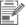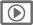Some patterns have been made up from ice block sticks.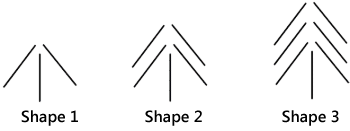How many ice cream sticks will be needed to make Shape 4 of this pattern?
Describe how to work out how many ice block sticks are needed to make any bigger shapes (for example Shape 10 or Shape 100).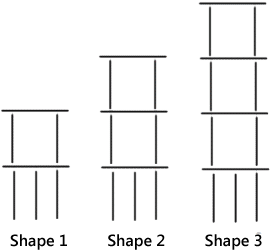How many ice cream sticks will be needed to make Shape 4 of this pattern?
Describe how to work out how many ice block sticks are needed to make any bigger shapes (for example Shape 10 or Shape 100).
This task can be completed with pen and paper or online (with SOME auto marking).
Level:
3
Curriculum info:
Students describe a functional rule for two spatial patterns.
Learning Progression Frameworks
This resource can provide evidence of learning associated with within the Mathematics Learning Progressions Frameworks.
 Y6 (03/2010) a) i) ii) 9 Accept functional and sequential descriptions of a rule.  Functional/direct rule: Two times the shape number plus one; Two for every number (shape) plus one for the bottom stick; Double the shape number and add one; Add one and go up in twos; 2n + 1 (where n = shape number); 3 + 2(n – 1); Other equivalent rules. Sequential/recursive rule*: Add 2 to the previous/last shape. Goes up in twos for example shape 6 will be shape 3 + 2 + 2 + 2 sticks; Goes up by one stick on each side and has one at the bottom to start. easy moderate b) i) ii) Accept functional and sequential descriptions of the rule. Accept functional and sequential descriptions of a rule as above for  3n + 4, 7 + 3(n – 1) or "add four each time" (respectively). easy moderate

NOTE: *Students who answered only referring to what happens to the previous shape need to be asked to clarify their rule either with an example or to identify the starting point (see Next steps).

Teaching and learning:

These questions have been phrased to encourage a functional (direct) rule which can be applied to find an nth term of a pattern, rather than a sequential rule which relies upon the previous term/s.

Click on the link to find out more about functional relationships or recursive strategies (Algebraic patterns concept map).

Diagnostic and formative information:

Strategies

About half the students successfully described a correct sequential rule. A number of students described how the spatial pattern grew, and several described a functional (direct) rule. The mean ability of students who described a functional rule was higher than students who described how the pattern grew or a sequential rule. A selection of students also included an example with their description of the rule for the pattern. A small group of students also drew the fourth shape.

 Common error Likely misconception a) i) b) i) 10 17 Error working out compensating for the intercept (initial term) Students have over compensated the intercept of the pattern. They have taken into account the increase of the pattern but have not correctly worked out the initial starting point (or intercept). Both errors are one more than the correct answer. b) i) 15 Students have not identified the part that changes and the initial starting point (intercept), i.e., if the extra stick at the base is ignored there are 5 storeys for shape 4 [3 times the shape number plus one, i.e., 3 × (n + 1)]. This could also be a misapplication of 3n + 1 (3 × one more than the shape number in the wrong sequence: adding one first). Students may have also used the rule 3n + 3 by missing the one vertical stick. a) i) 8 Students who described an incorrect/insufficient rule Students have ignored the intercept and used an incorrect rule (× 2 only). b) i) 13 Students use an incorrect rule for working out the next shape (3n + 1).

Examples of students' sufficient descriptions or explanations
Question a)
Functional/direct type rules (Curriculum level 3, early level 4)

• Shape 10 would be 2 × 10 = 20 plus one = 21; same method with 100.
• If I want find out how many for shape 20 I go 20 × 2 + 1 = 41.  So there are 41 sticks.
• To make shape 20 you will need one in the middle straight a 20 on the left on a diagonal and 20 on the right so you’ll have 41.
• You double the number then add 1 for the stick down the bottom.
• For the first one you add 3 and so on you only add 2.  So for shape 100 you just go 100 × 2 than add 1 and that’s your answer.
• Shape 10 would need 21 sticks because each shape has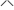how many times depending on what shape number they are: shape 249 would have 249s.  Each arrow has 2 sticks so multiply that by 2 and you get 498 + 1 (for the base) = 499 [correct application of rule, taking into account the intercept].
• You would go 2 × 10 or 100 + 1
• For each number, for example 10, you have 2 sticks on each side and one down the bottom.
• Start with the vertical stick and then whatever shape number you have been asked to make you just add that number off sticks. Find Shape 7:× 7 + 1.

Sequential/recursive type rules (Curriculum level 3)

• There was still 6 shapes between shape 4 and 9. So 6  × 2 = 12, because each shape will need another 2 sticks and plus 9 equals 21. [although this is not a generalisable rule, they have identified how earlier shapes can be used to work out the number of sticks for later shapes].
• Follow the pattern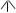to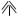to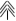every time the pattern gets 1bigger.
• Add two more sticks to the last one.
• Just add 2 every time.

Question b)

Functional/direct type rules

• You times the shape number by 3 and add 4.
• Key: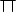- base.  Shape 100 would take 304 sticks because each shape hashow many times depending on what shape number they are, e.g., 333 would have 333s,= 3 sticks.
• 333 × 3 = 999 + 3 (base) = 1002 sticks.  [They add 3 not 4, but have shown that they know it is 4].
• You would go 3 × 10 or 100 + 4.

Sequential/recursive type rules

• Add 3 more sticks for each next shape.
• Follow the pattern each time up ais added.
• Each time you add 3 more sticks to the top.
• Shape one has 7 sticks and the next shape up needs three more added.

Examples of students' insufficient descriptions or explanations(some of these indicate a developing functional understanding)

Question a)

• Times by 2, because with every shape there is another 2 sticks being added onto that.
[This indicates a sequential understanding that they have tried to adapt to a functional rule - however they have ignored the intercept (plus 1)].
• Apart from the 3 sticks down the bottom there are the amount of storeys with the number of the shape that all have the same amount of sticks per 1 storey.
[This generalising could be the start of developing a functional understanding of the pattern].
• For Shape 10 they will need 15.
• Seven plus (two) equals nine.
• The number of the shape is the same amount of sticks you just add one. For instance number 100 will have 101 sticks.
• Just times it.

Question b)

• Times it by 4.
• You just keep adding 3 after you have done shape I.  But for shape 1 you have 3 sticks down the bottom and 4 on top.
• Times by 3.  Because in shape one it has made the base for the next square added onto that therefore adding 3 stick every time.
• If it is shape 6 I go 3 × 7 = 21 so I add 1 to the shape number and times it by 3. [(n + 1)  ×  3]
• To make shape 10 you will need to have three at the bottom pointing up a then ten stories high.
• You need 3 sticks for the bottom and 4 sticks for a square top so you times 8 by whatever number.
• For shape 10 they will need 34.
• Shape 10 would be 4 × 10 = 40 - 1 = 39 same method with for 100.
Next steps:

Not able to identify the number of sticks for the next shape
Students who could not identify the number of sticks needed to make "the next shape" could be asked to identify the number of sticks in earlier shapes (1, 2 , and 3) and then asked how many sticks might be needed for the next shape.  The resource, Continue the shape patterns looks at exploring the next shapes in growing spatial patterns. Students could also be given opportunity to construct growing patterns and explore the increase and the starting point [see Making stick patterns, Making triangle patterns and Building square patterns].

Cannot adequately write a rule for the pattern
For students who could not write a rule to describe the growing pattern get them to explore situations continuing or constructing patterns (see above). Once they can continue a pattern encourage them to orally describe what is happening to the pattern and to identify the parts that are increasing and what stays constant. If they can reasonably successfully continue the pattern but say "I just saw it" or something similar probe just what it is that they saw. If they can articulate it, encourage them to then write it down in words. Students could explore identifying rules for spatial growing patterns in Match patterns and also creating their own patterns and rules as an extension of that resource.

Students who described an incorrect/insufficient rule
Encourage the student to identify what is happening from one shape to the next to develop a sequential idea of how the pattern grows.  The resources Pyramid pattern and Stacking cans look at developing sequential rules for growing spatial patterns at Level 3. Students could share their rules for the patterns in small groups and check that each others' rules work for all members of the pattern (see Providing a sufficient explanation-Peer assessment below). Once students can identify the sequential rule they could look at developing a functional rule (see Students who described a sequential rule below).

Error working out compensating for the intercept (initial term)
Get students to create more shapes of each pattern. Ask, "Does Shape 6 have twice as many sticks as Shape 3?" or "Does Shape 3 have three times as many as Shape 1?" For question a) remove the vertical stick of each shape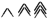and ask how the pattern grows and compare this to the pattern with the vertical stick. The vertical stick is the intercept. For question b) take away the 3 vertical sticks and bottom horizontal of each shape and ask how the pattern grows.  Then compare to the pattern given in this resource. Have students explore and describe the difference.

This should help students see how the intercept influences the pattern. For example in question a) the first shape has 1 + 2 sticks, the second 1 + 2 + 2, so the 1 is the initial term of an arithmetic progression (as well as the intercept) and the 2 is the common difference (or increase) - which becomes the number that n is multiplied by in the functional rule for the pattern. Similarly, for b) the initial term is 4 and the common difference is 3, so for shape 5 there are 4 + 3 + 3 + 3 + 3 + 3 = 4 + 5  × 3.

Students who described a sequential rule
For students who described a sequential rule, ask them how they might find a rule that would work for any given number, e.g., How many sticks for shape 100? Trying to use the sequential rule should be unwieldy and the need for a functional rule can become evident.  It can also mean that students do not develop an understanding about patterns with an intercept. Additionally, as students apply their sequential rule they may see the connection between the number they are adding on to the previous shape and the number that n (shape number) is multiplied by in the functional rule.

Students who described a functional rule with some errors

For students who described a functional rule, but made some errors, encourage them to share and explain their rule, and check that the rule works for all members of the pattern - not just the first two. Using shape numbers like 10 and 100 make it easier to see the relationship in the pattern,
e.g., 3n + 4, for n = 100 is 304. Encourage students to include examples to help clarify their description of the pattern. A small group discussion should provide peer support to re-check the completeness and accuracy of their rule.

Providing a sufficient explanation-Peer assessment
Many of the students gave explanations that were just sufficient, but did not fully describe or explain how they worked it out. These students could share their explanation (or explanations from this resource) in a group to identify details and clarify ideas that are needed in their explanations.  Students could develop criteria for an explanation and do some peer assessments.
They could also have a "sufficient" explanation modelled and made explicit to them (by other classmates or the teacher). A criteria* of what makes an explanation sufficient might be:

1. specific details
2. appropriate use of or reference to examples
3. justification